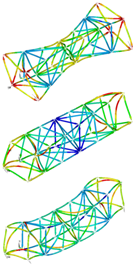Ansys®-Matlab® Interface

The AdaptroSim® ANSYS®-MATLAB® Interface combines the possibilities of the FE-tool ANSYS® with MATLAB/Simulink®. With the help of AdaptroSim® ANSYS®-MATLAB® Interface toolbox ANSYS® models can be reduced in various ways and co-simulations can be implemented. Specifically, the following options are available in the AdaptroSim ANSYS®-MATLAB® Interface:

 ANSYS® Workbench-Wizard for creating reduced structural modelsANSYS® Classic routines for creating reduced structural modelsControlling the ANSYS® simulation from MATLAB® by using APDL Batch Controller and APDL script parametrizer, e.g. for optimization tasks.The following binary ANSYS® files can be imported and processed in MATLAB®:Full: SystemmatrizenRST: ANSYS® ResultsSUB: System matrices of reduced modelsTCMS: Transformation matrix reduced modelsMode: Modal dataImporting ANSYS® – SPMWRITE dataModel reduction Krylov subspace method in MATLAB®Following blocks and functions from the AdaptroSim® Ansys®-Matlab® Interface can be used:

Matlab®

Ansys

Classes

ma_ansys package

ma_ansys.binary – Class representing general Ansys binary file

ma_ansys.emat Structured representation of ansys binary *.emat files

ma_ansys.full – Class representing Ansys *.full-Files Mapping of the degrees of freedom of the system

ma_ansys.cms – Class representing modal reduced model *.cms files

ma_ansys.modal_data – A wrapper class for Ansys modal results (*.rst)

ma_ansys.result – Class representing Ansys result *.rst files

ma_ansys.sub – Class representing Ansys *.sub substructure files

ma_ansys.mode – Class representing modal data from Ansys *.mode files

ma_ansys.tcms – Class representing the CMS transformration matrix *.tcms file

ma_ansys.AnsysFull – A wrapper class for ma_ansys.full implementing the ma_common.SecondOrderSystem interface

ma_ansys.AnsysModalResult – A wrapper class for ma_ansys.result implementing ma_common.ModalSolution

ma_ansys.AnsysResultData – A wrapper class for ma_ansys.result implementing ma_common.SignalName of the result file

ma_ansys.AnsysSub – A wrapper class for ma_ansys.sub implementing the ma_common.SecondOrderSystem interface

ma_ansys.runAnsysScriptFile – Method to execute APDL-Code from Ansys in Matlab

ma_ansys.writeAnsysScript – Method to create and assemble APDL-Code within Matlab

Plug-in for Ansys

cms_wizard – Wizard for generating reduced models within ANSYS Workbench Mechanical

Common Matlab® functions

ma_import_fft_analyser_data – Imports data table and header from ASCII

ma_fft_analyser_frf_plot – Returns the FRF, recorded by an FFT-analyser

ma_common package

ma_common.Channel – Represents a data channel (i.e. sensor) transporting system information

ma_common.ContinousTimeStateSpace – Represents a continuous-time state-space model

ma_common.DiscreteTimeStateSpace – Represents a discrete-time state-space model

ma_common.Frf – Abstract interface definition, defining frequency response function of a linear-time-invariant system

ma_common.GenericSignal – Represents a signal with its channels and units of the abscissa and ordinate

ma_common.GenericFrf – Represents the frequency response function of a system

ma_common.Geometry – Class definition for a geometry consisting of nodes and edges

ma_common.ModalSystem – Represents system dynamics described by means of modal vectors, modal frequencies and modal damping

ma_common.Node – Represents a node in a Cartesian coordinate system by its x-, y- and z-coordinates

ma_common.SecondOrderSystem – Describes a second order system using a second order linear differential equation

ma_common.Signal – Signal data container with channels that share a common abscissa

ma_common.StateSpace – Represents the time-domain state-space model of a system

ma_common.System – Represents a system with its input- and output-channels

ma_common.Unit – Represents units by exponents and factors

Sensors

AccelerationSensor – Implements a simple acceleration sensor

DisplacementSensor – Implements a simple displacement sensor

ForceSensor – Implements a simple force sensor

VelocitySensor – Implements a simple velocity sensor

Compatibility Consideration

System requirements: AdaptroSim requires a Windows 64bit environment, a working copy of Matlab 2014b or later installed and you have a C compiler setup via mex -setup c command as described here.